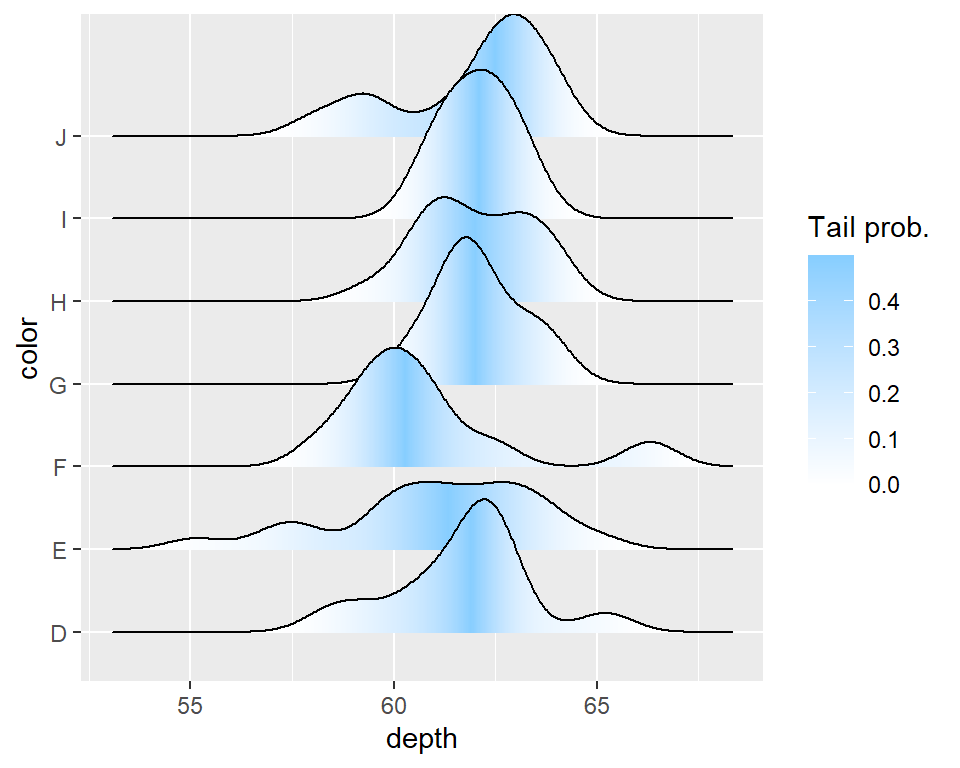# Ridgeline plot in ggplot2 with ggridges

Claus O. Wilke

## Sample data

For this tutorial we are going to use a subset of the `diamonds` data set from `ggplot2` package.

``````# install.packages("ggplot2")
library(ggplot2)

# Sample data
df <- diamonds[1:100, c("color", "depth")]``````

## Ridgeline plots (joy plots) with `geom_density_ridges`

The `geom_density_ridges` function from the `ggridges` package allows creating a ridgeline visualization. Given a numerical variable (`depth`) and a categorical variable (`color`) a density estimation of the data will be calculated and displayed for each group.

``````# install.packages("ggridges")
library(ggridges)
# install.packages("ggplot2")
library(ggplot2)

ggplot(df, aes(x = depth, y = color)) +
geom_density_ridges()``````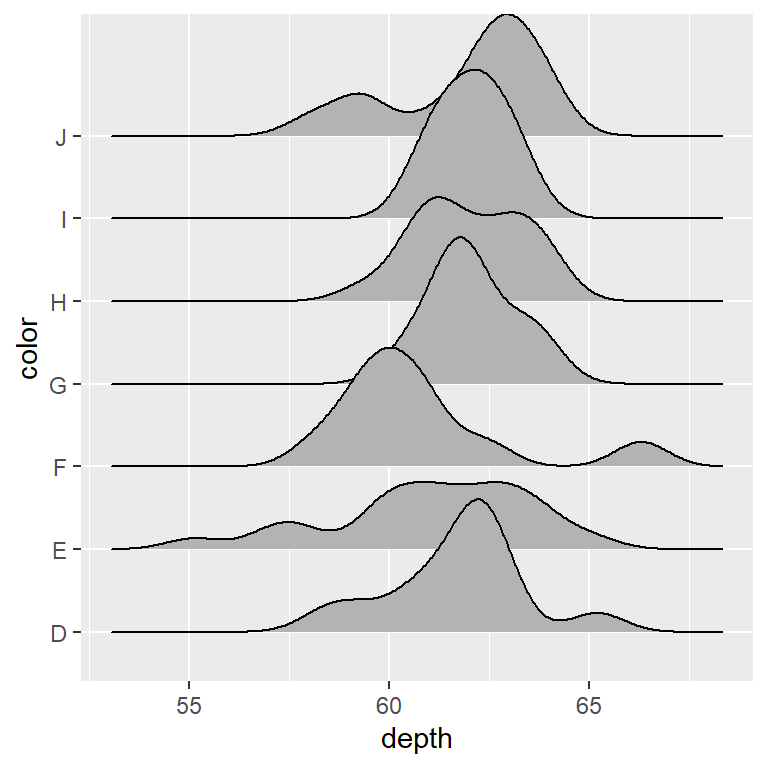geom_density_ridges2

Note that there is an equivalent function named `geom_density_ridges2` which uses closed polygons to display the density estimates, so the line will also appear at the bottom of each density estimation.

``````# install.packages("ggridges")
library(ggridges)
# install.packages("ggplot2")
library(ggplot2)

ggplot(df, aes(x = depth, y = color)) +
geom_density_ridges2()``````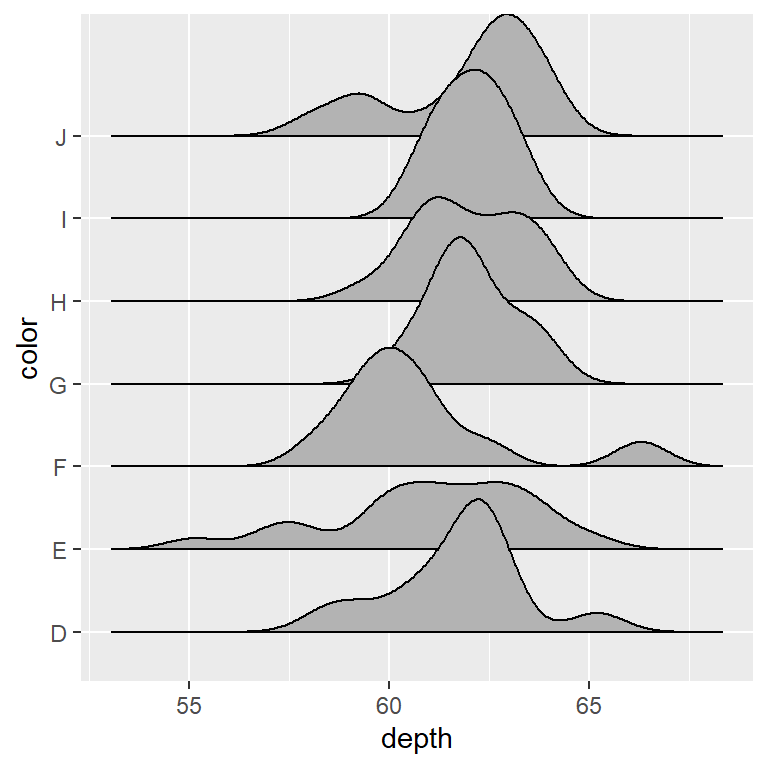Cut the trailing tails

The `rel_min_height` argument of the function can be used to cut the trailing tails. You will need to fine tune the value depending on your data.

``````# install.packages("ggridges")
library(ggridges)
# install.packages("ggplot2")
library(ggplot2)

ggplot(df, aes(x = depth, y = color)) +
geom_density_ridges(rel_min_height = 0.005)``````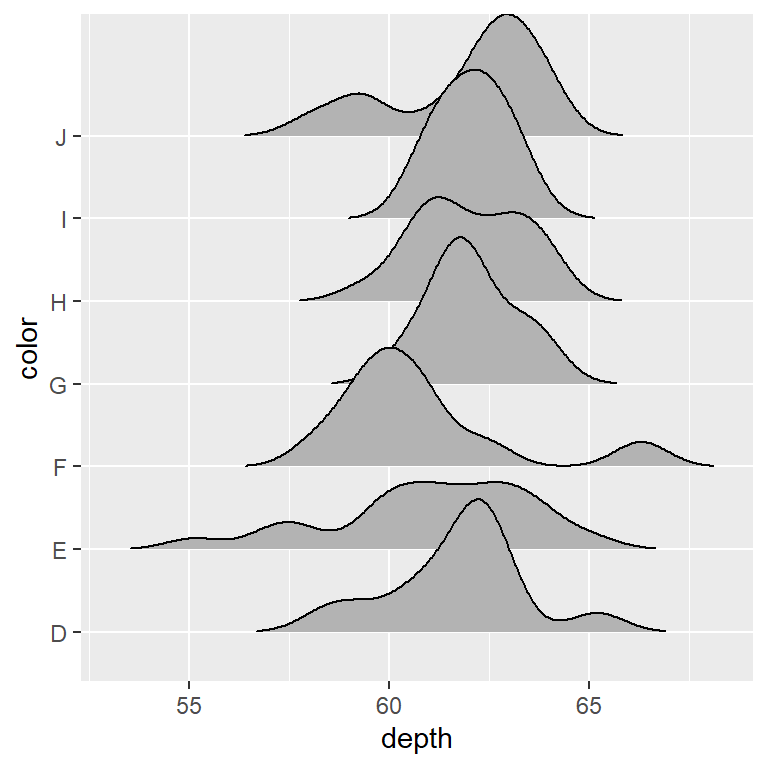Scale

In addition, the `scale` argument controls the scaling of the ridgelines relative to the spacing between them.

``````# install.packages("ggridges")
library(ggridges)
# install.packages("ggplot2")
library(ggplot2)

ggplot(df, aes(x = depth, y = color)) +
geom_density_ridges(scale = 3)``````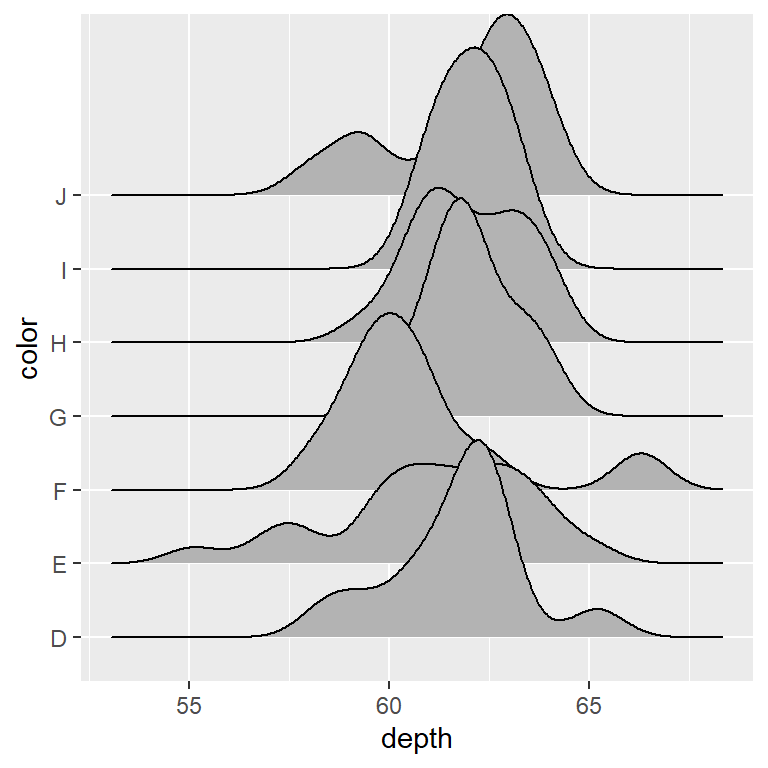Alternative stats

The `stat` argument can be used to select the statistical transformation to be used.

``````# install.packages("ggridges")
library(ggridges)
# install.packages("ggplot2")
library(ggplot2)

ggplot(df, aes(x = depth, y = color)) +
geom_density_ridges(stat = "binline",
bins = 20, draw_baseline = FALSE)``````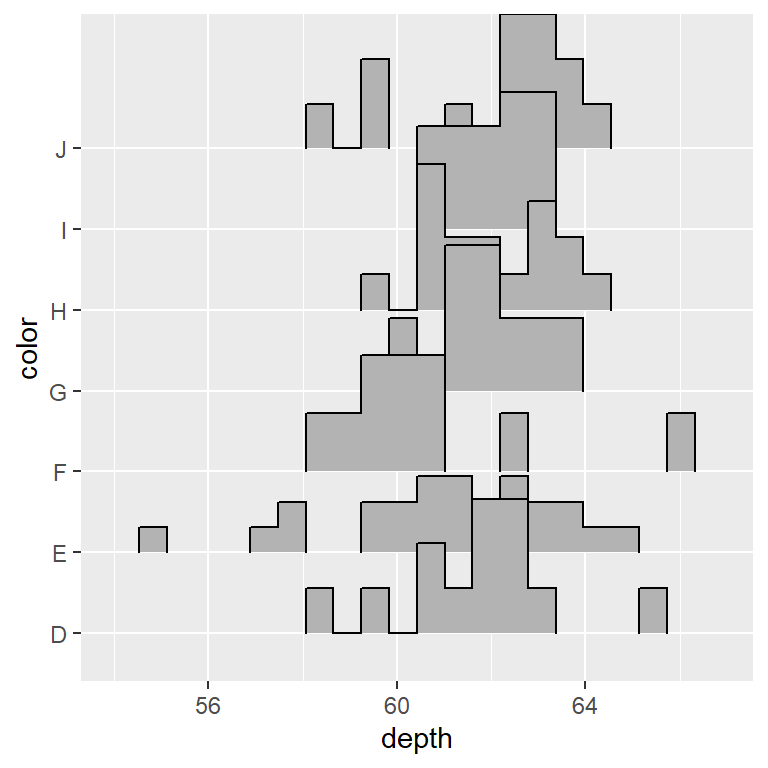## Color customization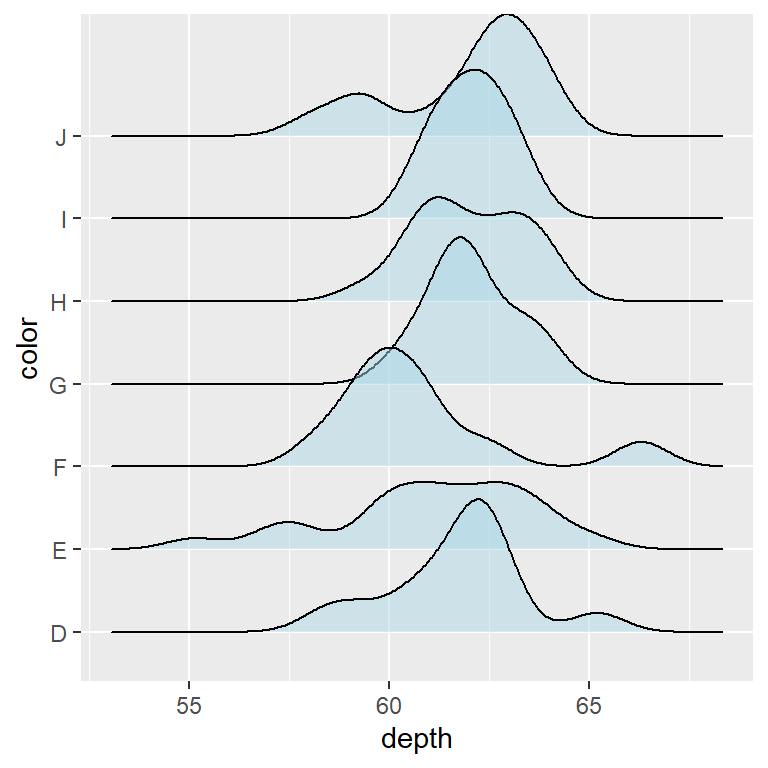Fill color and transparency

The default gray color of the ridgelines can be changed with the `fill` argument of the `geom_density_ridges` function. Note that you can also specify a level of transparency with `alpha`.

``````# install.packages("ggridges")
library(ggridges)
# install.packages("ggplot2")
library(ggplot2)

ggplot(df, aes(x = depth, y = color)) +
geom_density_ridges(fill = "lightblue", alpha = 0.5)``````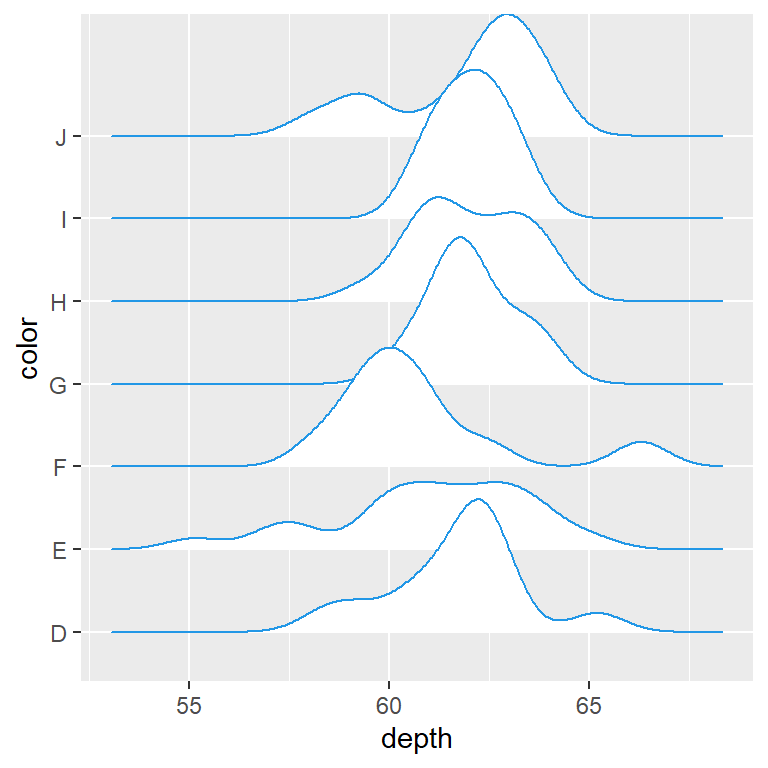Border color

The `color` argument of the function controls the color of the lines. As in other plots you can also change the line type and the width of the lines.

``````# install.packages("ggridges")
library(ggridges)
# install.packages("ggplot2")
library(ggplot2)

ggplot(df, aes(x = depth, y = color)) +
geom_density_ridges(fill = "white",
color = 4,
linetype = 1,
lwd = 0.5)``````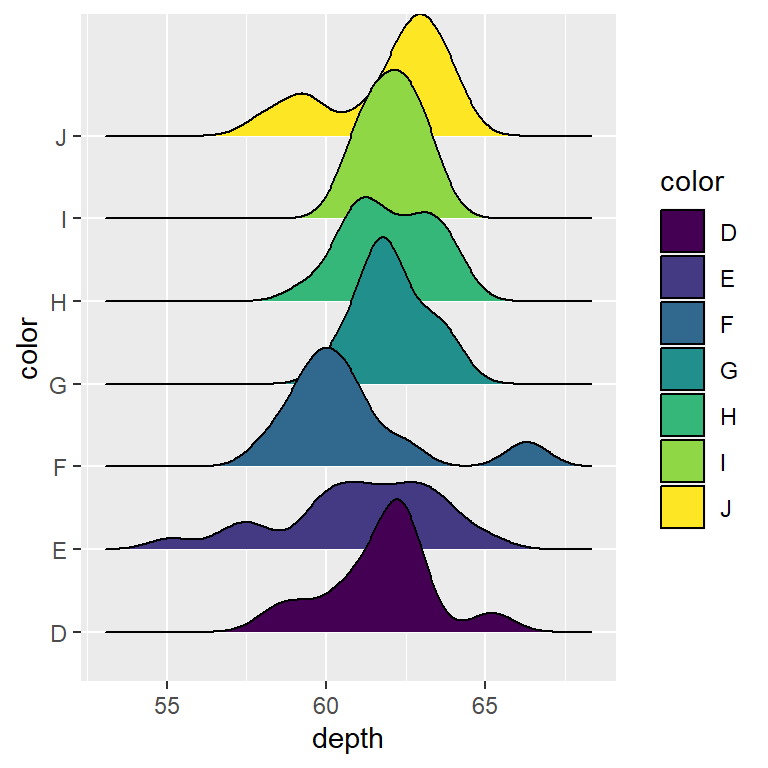Color based on group

You can also fill the densities based on the categorical variable, passing it to the `fill` argument of `aes`. The color palette can be changed with `scale_fill_manual`, for instance.

``````# install.packages("ggridges")
library(ggridges)
# install.packages("ggplot2")
library(ggplot2)

ggplot(df, aes(x = depth, y = color, fill = color)) +
geom_density_ridges()``````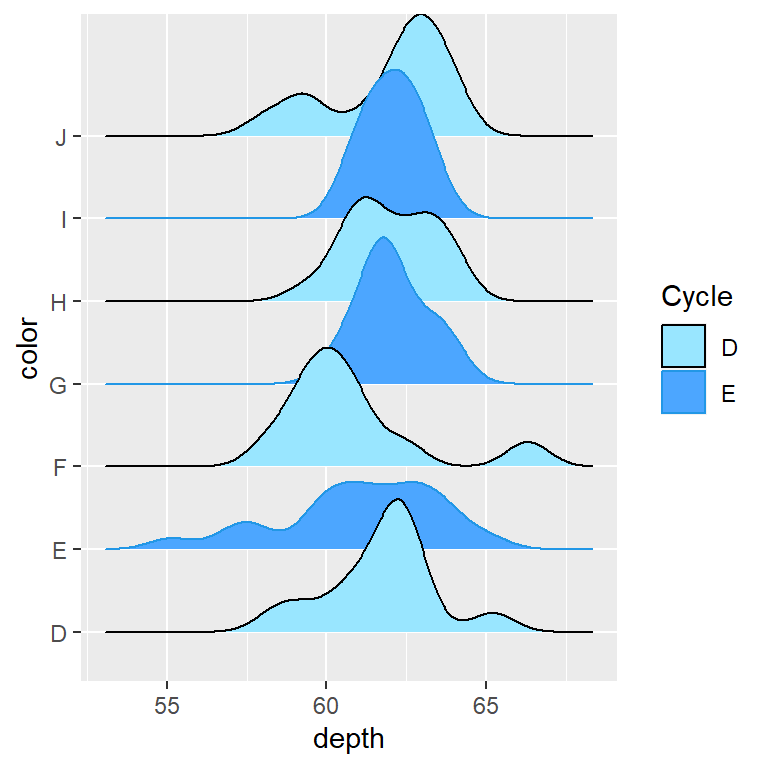Cyclical color scales

The `scale_fill_cyclical` and `scale_color_cyclical` functions can be used to add cyclical fill and border colors to the density estimations.

``````# install.packages("ggridges")
library(ggridges)
# install.packages("ggplot2")
library(ggplot2)

ggplot(df, aes(x = depth, y = color, fill = color, color = color)) +
geom_density_ridges() +
scale_fill_cyclical(name = "Cycle", guide = "legend",
values = c("#99E6FF", "#4CA6FF")) +
scale_color_cyclical(name = "Cycle", guide = "legend",
values = c(1, 4))``````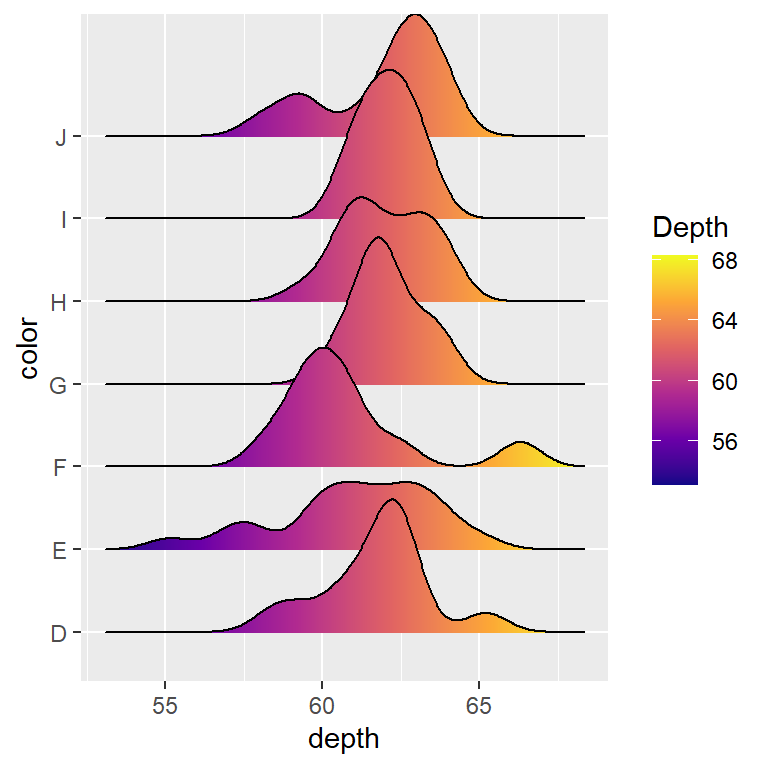You can pass `stat(x)` or `factor(stat(x))` to the `fill` argument of `aes` and use `geom_density_ridges_gradient` and a continuous fill color scale to fill each ridgeline with a gradient.

``````# install.packages("ggridges")
library(ggridges)
# install.packages("ggplot2")
library(ggplot2)

ggplot(df, aes(x = depth, y = color, fill = stat(x))) +
scale_fill_viridis_c(name = "Depth", option = "C")``````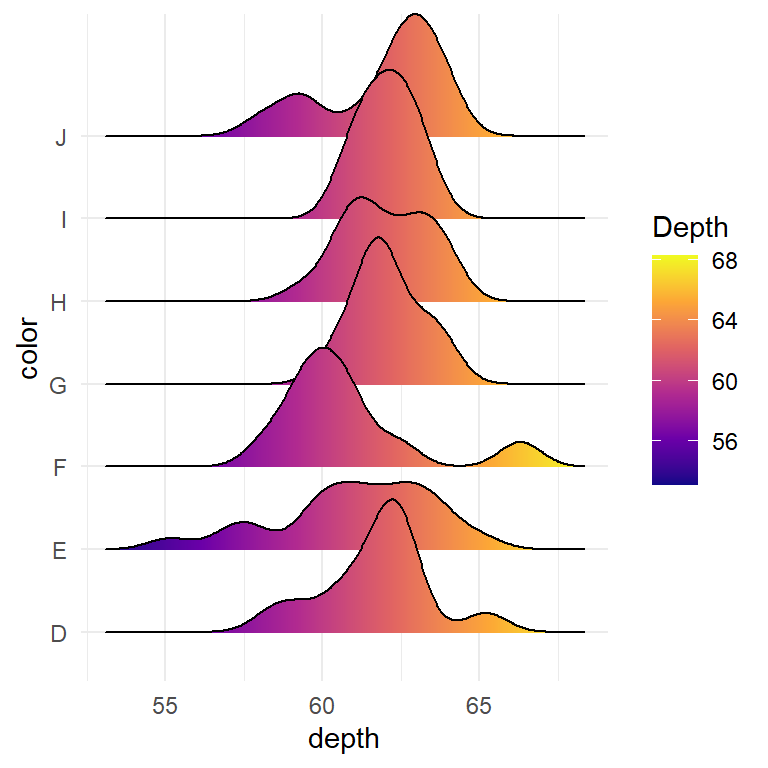Change the theme

Note that you can also change the ggplot2 theme to modify the appearance of the plot. The `ggridges` package provides the `theme_ridges` theme.

``````# install.packages("ggridges")
library(ggridges)
# install.packages("ggplot2")
library(ggplot2)

ggplot(df, aes(x = depth, y = color, fill = stat(x))) +
scale_fill_viridis_c(name = "Depth", option = "C") +
coord_cartesian(clip = "off") + # To avoid cut off
theme_minimal()``````

## Adding quantiles and probabilities with `stat_density_ridges`

If you use `stat_density_ridges` instead of `geom_density_ridges` and set the `quantile_lines` argument to `TRUE` the quantiles will be calculated and displayed for each density.

``````# install.packages("ggridges")
library(ggridges)
# install.packages("ggplot2")
library(ggplot2)

ggplot(df, aes(x = depth, y = color)) +
stat_density_ridges(quantile_lines = TRUE, alpha = 0.75)``````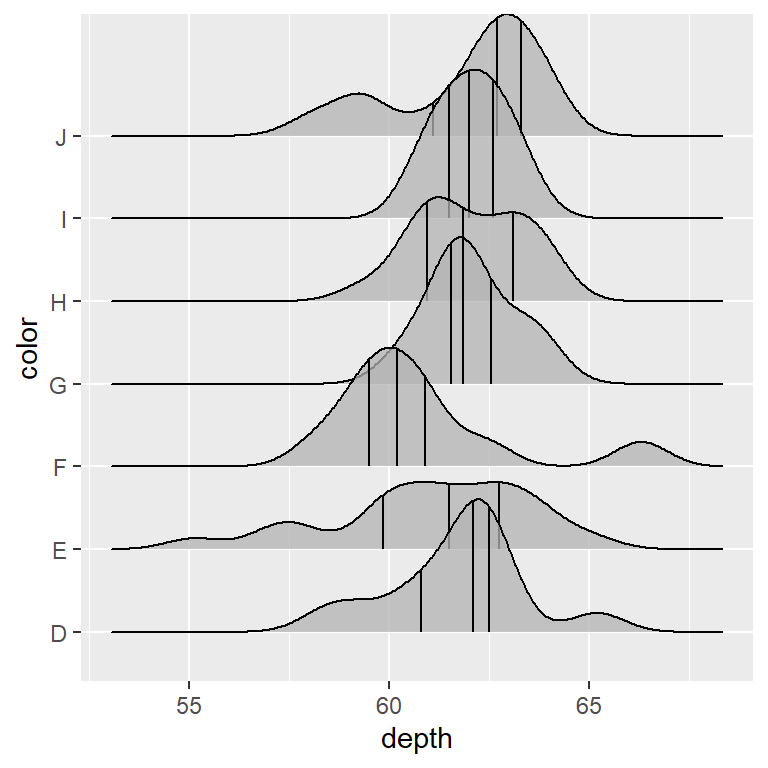Number of quantiles

The `quantiles` argument controls which or how how many quantiles are displayed. If you pass an integer the data will be cut into that many equal quantiles.

``````# install.packages("ggridges")
library(ggridges)
# install.packages("ggplot2")
library(ggplot2)

ggplot(df, aes(x = depth, y = color)) +
stat_density_ridges(quantile_lines = TRUE, alpha = 0.75,
quantiles = 2)``````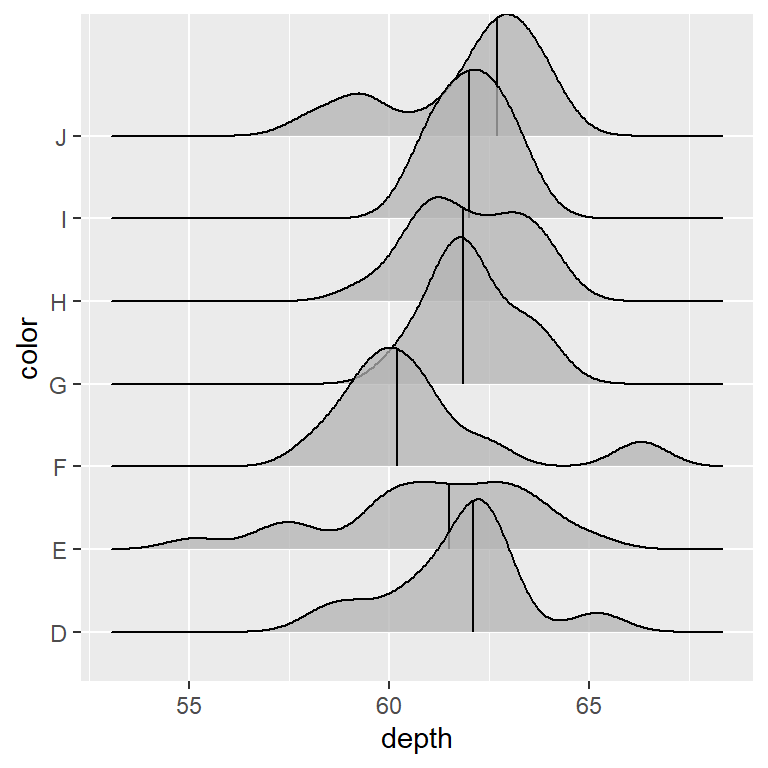Specify the quantiles

If you pass a value or a vector of values between 0 and 1 to the `quantiles` argument those quantiles will be calculated and displayed.

``````# install.packages("ggridges")
library(ggridges)
# install.packages("ggplot2")
library(ggplot2)

ggplot(df, aes(x = depth, y = color)) +
stat_density_ridges(quantile_lines = TRUE, alpha = 0.75,
quantiles = c(0.05, 0.5, 0.95))``````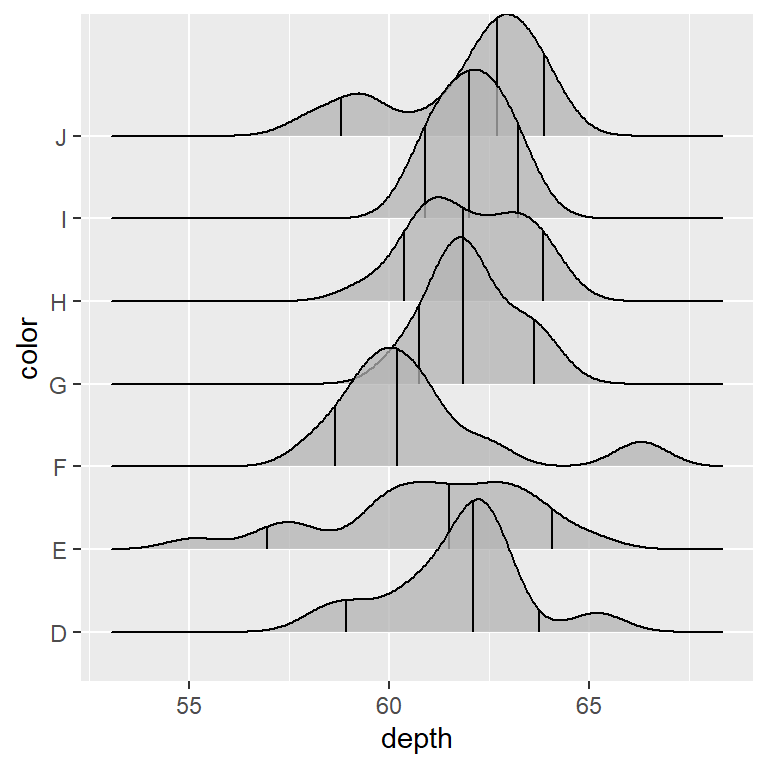Color by quantile

Passing `stat(quantile)` to `fill` and setting `calc_ecdf` and `geom = "density_ridges_gradient"` each quantiles will be filled with a different color. Note that if you set `quantile_lines = TRUE` the vertical lines for each quantile will be drawn.

``````# install.packages("ggridges")
library(ggridges)
# install.packages("ggplot2")
library(ggplot2)

ggplot(df, aes(x = depth, y = color, fill = stat(quantile))) +
stat_density_ridges(quantile_lines = FALSE,
calc_ecdf = TRUE,
scale_fill_brewer(name = "")``````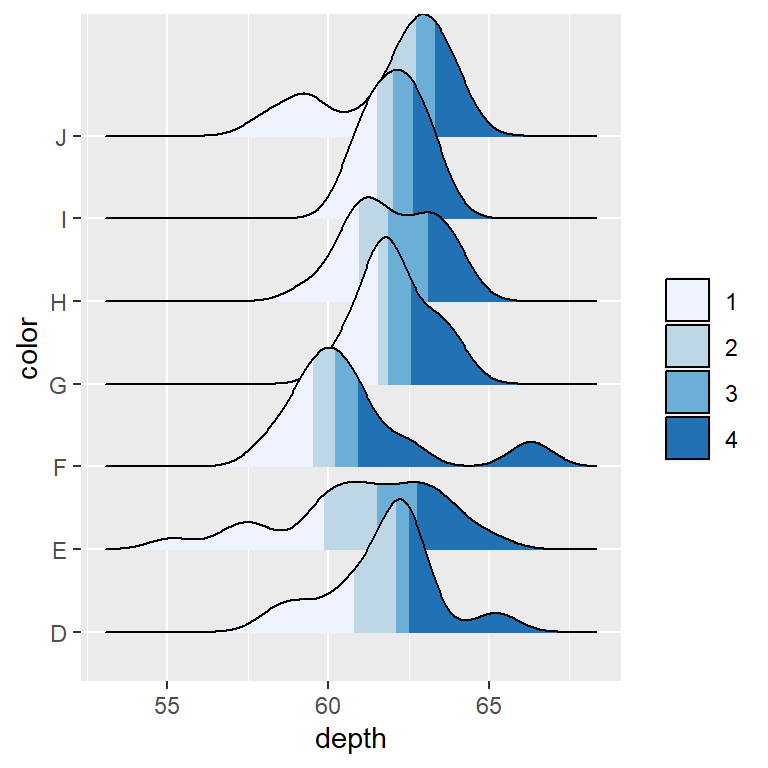Highlight the tails of the distribution

The same approach described above can be used to highlight the tails of the distributions.

``````# install.packages("ggridges")
library(ggridges)
# install.packages("ggplot2")
library(ggplot2)

ggplot(df, aes(x = depth, y = color, fill = stat(quantile))) +
stat_density_ridges(quantile_lines = TRUE,
calc_ecdf = TRUE,
quantiles = c(0.05, 0.95)) +
scale_fill_manual(name = "Prob.", values = c("#E2FFF2", "white", "#B0E0E6"),
labels = c("(0, 5%]", "(5%, 95%]", "(95%, 1]"))``````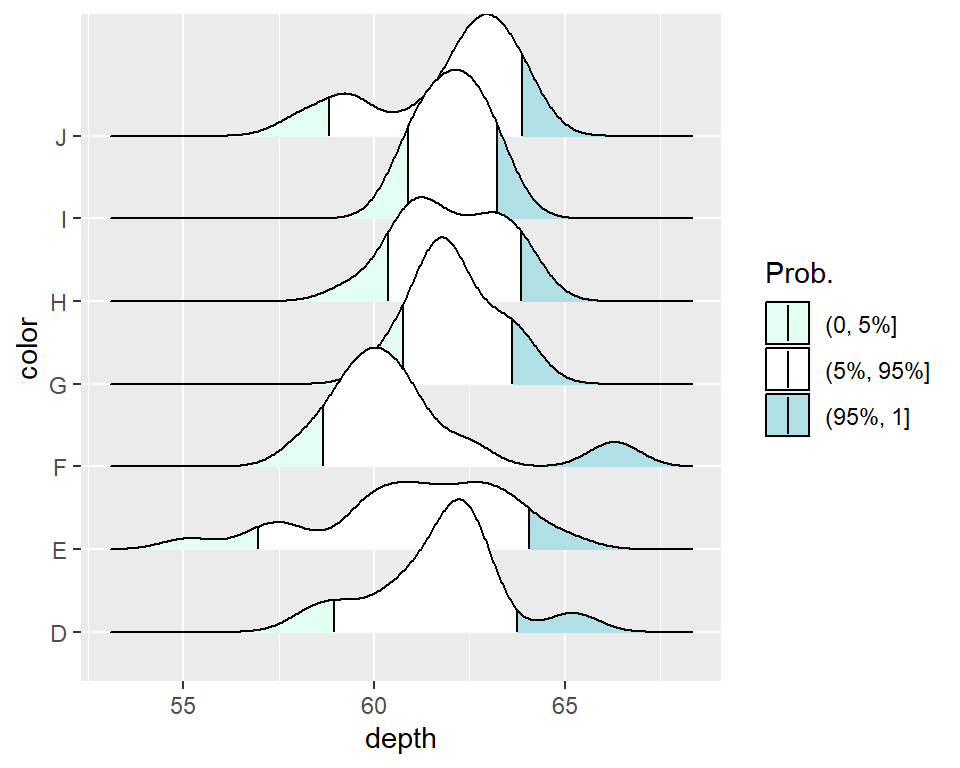Mapping the tails probabilities onto color

Similarly, using `stat(ecdf)` it is possible to add a gradient to the densities displaying the tail probabilities.

``````# install.packages("ggridges")
library(ggridges)
# install.packages("ggplot2")
library(ggplot2)

ggplot(df, aes(depth, y = color,
fill = 0.5 - abs(0.5 - stat(ecdf)))) +
stat_density_ridges(geom = "density_ridges_gradient", calc_ecdf = TRUE) +
scale_fill_gradient(low = "white", high = "#87CEFF",
name = "Tail prob.")``````